Courses

# Test: Strain Energy & Resilience Level - 2

## 10 Questions MCQ Test Solid Mechanics | Test: Strain Energy & Resilience Level - 2

Description
This mock test of Test: Strain Energy & Resilience Level - 2 for Mechanical Engineering helps you for every Mechanical Engineering entrance exam. This contains 10 Multiple Choice Questions for Mechanical Engineering Test: Strain Energy & Resilience Level - 2 (mcq) to study with solutions a complete question bank. The solved questions answers in this Test: Strain Energy & Resilience Level - 2 quiz give you a good mix of easy questions and tough questions. Mechanical Engineering students definitely take this Test: Strain Energy & Resilience Level - 2 exercise for a better result in the exam. You can find other Test: Strain Energy & Resilience Level - 2 extra questions, long questions & short questions for Mechanical Engineering on EduRev as well by searching above.
QUESTION: 1

### Consider the following statements.(P) Hardness is the resistance of a material to indentation.(Q) Elastic modulus is a measure of ductility.(R) Deflection depends on stiffness.(S) The total area under the stress-strain curve is a measure of resilience.Among the above statements, the correct ones are

Solution: Percentage elongation is a measure of ductility. The total area under the stress-strain curve is a measure of modulus of toughness.

QUESTION: 2

Solution:
QUESTION: 3

### Which one of the following statements is correct? The work done in stretching an elastic string varies

Solution: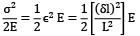QUESTION: 4

The maximum stress induced in a body if the load ‘P’ is applied with impact upon an area of cross section ‘A’ is (When h = Height through which the load falls, E = Modulus of rigidity, L = Length of the body)

Solution:
QUESTION: 5

Which of the following gives the correct expression for strain energy stored in a beam of length L and of uniform cross section having moment of inertia ‘I’ and subjected to constant bending moment M?

Solution:
QUESTION: 6

A bar having length L and uniform cross section with area A is subjected to both tensile force P and torque T. If G is the shear modulus and E is the Young’s modulus, the internal strain energy stored in the bar is

Solution: Internal strain energy

=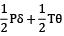=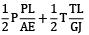QUESTION: 7

An axial load of 100kN is applied onto a bar of length 3 m and cross-sectional area of 100 mm2. If E = 200 kN/mm2 the total strain energy in the bar is equal to

Solution: Axial load, P = 100 × 103N

Length, L = 3000 mm 2

Cross section area, A = 100 mm E = 200 × 103 N/mm2

U =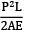=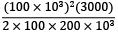=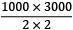= 750000 N­mm

QUESTION: 8

A circular bar L (long) in m and d (diameter) in m is subjected to tensile force of F in kN. Then the strain energy, U will be (Where, E is the modulus of elasticity in kN.m2)

Solution:

℧ =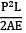=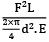=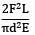QUESTION: 9

The strain energy in bar of 20 mm diameter, 1.0 m length, and young’s modulus 200.0 GPa under an axial force of 100.0 N is

Solution: Diameter, d = 20 mm

Length, L = 1000 mm

E = 200 × 103 MPa

P = 100N

U =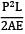=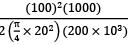=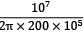U = 0.0796 N­mm

QUESTION: 10

If σ and E for a body of volume 2 × 105 mm3 are 10 N/mm2 and 1 × 105 N/mm2, Resilience of the body is

Solution: σ = 10N/mm2

E = 1 × 105N/mm2

V = 2 × 105N/mm3

U =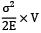U =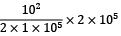= 100 N­mm